# Class 9 Maths Extra Questions for Linear equations in two variable

Given below are Chapter 4 Linear equations in two variables class 9 extra questions and linear equations in two variables class 9 important questions
(a) Concepts questions
(b) Calculation problems
(c) Multiple choice questions
(d) tough problems
(e) word problems

Question 1
Ravish tells his daughter Aarushi, ‘ Seven years ago, I was seven times as old as you were then also, three years from now, I shall be three times as old as you will be.’ If the present ages of Aarushi and Ravish are x and y years respectively, represent this situation algebraically as well as graphically.
Solution
First condition
y-7 = 7(x-7)
or y = 7x -42
Second Condition
y+3 = 3(x+3)
or y= 3x + 6
Now we can draw the graph of both the linear equation by choosing points on x-axis and y -axis i.e by firt putting x=0 ,getting the y -coordinate and then putting y=0 and getting the x-coordinate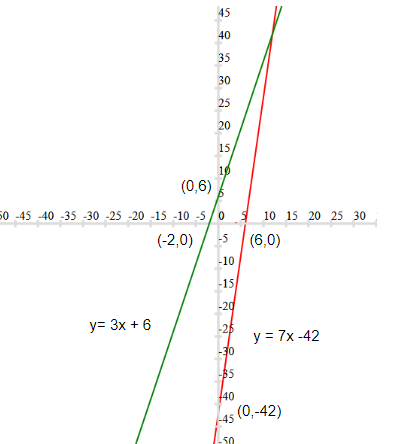Question 2
Two students of a College contribute to charity. The contribution of A is 2/5 of contribution of B. Write a linear equation according to the above statement and draw the graph for the linear equation.
Solution
Let y be the contribution of A and x be the contribution of B
Then as per question
$y= \frac {2}{5} x$
$5y=2x$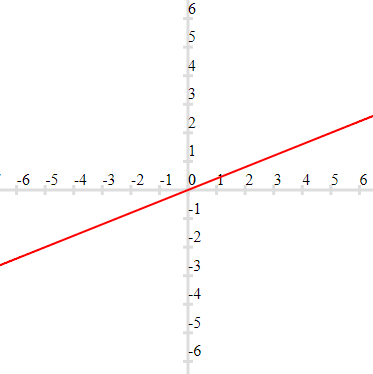Question 3
A and B are friends. A is elder to B by 5 years. B’s sister C is half the age of B while A’s father D is 8 years older than twice the age of B. If the present age of D is 48 years, find the present ages of A, B and C.
Solution
Let x be the age of B
Let y be the age of A
Now from question
y=x + 5
Now also
D Age = 2x + 8
Now D age =48,So
48=2x+8
x= 20 years
Hence y = 25 years
Now C's Age is half the age of B. Therefore C's Age will be 10 years

Question 4
Cost of 5 kg apples and 2 kg oranges is Rs. 330. Let the cost of 1 kg apples be Rs. x and that of 1 kg is Rs. y. Write the given data in the form of linear equation in two variables. Also , represent it graphically.
Solution
5x + 2y=330Question 5
The parking charge for vehicles in super Delhi Metro is Rs 20 for first two hrs and Rs. 10 for subsequent hr. Assume total parking time to be x hrs. (where x>= 2 ) and total parking charge as y . Write the linear equation for above relation and draw graph. Find the parking Charges for 5 hrs from Graph
Solution
y = 20 + 10(x-2)
y= 10x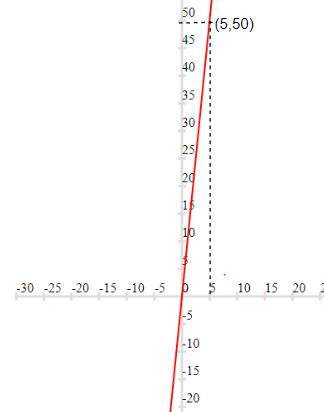We can observe that parking charge for 5 hours is Rs 50

Question 6
P and Q are two students of IXth class of DPS school. Together, they contributed Rs 100 towards the old age home to help the old persons.
Write a linear equation which satisfies the given data and draw the graph. What values are depicted in their plan?

Question 7
Mrs.Agarwak lost her purse containing 50 rupee and 100 Rupee notes amount to Rs 1500 in a shop. Next day shopkeeper found the purse during dusting. He immediately went to Mrs. Agarwal’s house and returned the purse and rupees Mrs.Agarwal appreciates the shopkeeper for his act.
Represent this situation as an equation and draw the graph. What value do you learn from the shopkeeper’s act?

Question 8
In an election, a good candidate may lose because 40% of voters do not cast their votes. Form an equation and draw the graph with data. From the graph find,
(a)The total no. of voters, if 720 voters cast their votes.
(b)The number of votes cast, if the total number of voters are 1000
(c) What message did you cast from above information?
Solution
Let x be the total number of voters and y be the number of voter who cast their votes
Then as per question
y = 60% x
$y = \frac {60x}{100}$
$10y = 6x$
The graph can be drawn with the help of two points (0,0) and (400,240)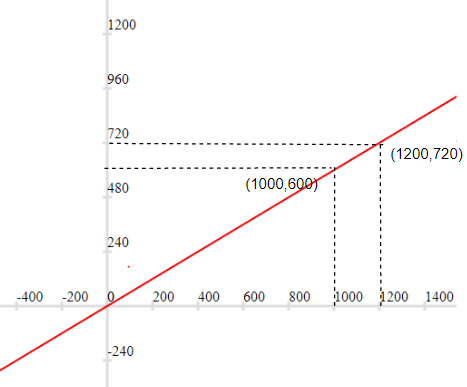Now from the graph, it is clear that total of no. of voters is 1200 when 720 voters cast their votes
And The number of votes cast is 600 when total number of voters are 1000
The message we get that large number of people does not cast their vote and do not participate in selection of good candidate

Question 9
The cost of Video game is twice the cost of teddy bear. Write a linear equation in two variables to represent this statement.
Solution
Let x be the cost of teddy bear and y be the cost of Video game
$y=2x$

Question 10
Thrice the cost of a kettle is equal to five times the cost of an oven. Write a linear equation in two variables to represent this statement.
Solution
Let x be the cost of oven and y be the cost of kettle
$3y=5x$

Question 11
Draw the graph of the equation y = mx + c for m = 3 and c = -1 (a straight line in Cartesian plane). Read from the graph the value of y when x = 2.
Solution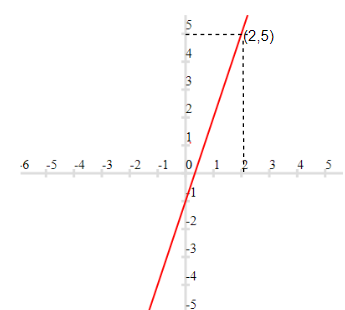Question 12
If x is the number of hours a laborer is on work and y is his wages in rupees, then y = 4x + 3. Draw the work wages graph of this equation. From the graph, find the wages of a laborer who puts in 4 hours of work.

Question 13
Find whether (a,a) lies on the lines $y - x = 0$ or not.
Solution
By putting the values x=a and y=a
$a -a=0$
$0=0$
So, point (a,a) lie on the line

Question 14
Find the point on x axis from where graph of linear equation x - 5y = 3 will pass.
Solution
Let the point on x -axis is (a,0)
Putting x=a and y=0
$a -5 \times 0 =3$
$a=3$
So the point is (3,0)

Question 15
if ax + 3y = 25 and y = 1 find value of x
Solution
ax + 3y = 25
Putting y=1
$ax + 3 =25$
$ax=22$
$x = \frac {22}{a}$

Question 16
When a stone is falling from a mountain its initial velocity is zero and the acceleration due to gravity is 9.8m/s2. Velocity of the stone is given by v = 9.8t.Draw its graph and find the velocity of stone after 4 secs?

Question 17
A linear equation in two variables is of the form
$px + qy + r = 0$, where
(a) $p \ne 0$, $q \ne 0$
(b) $p = 0$, $q \ne 0$
(c) $p \ne 0$, $q = 0$
(d) $p = 0$, $r = 0$
Solution

Question 18
The perimeter of a rectangle is 80 m. If the length of the field is decreased by 2 m and breadth increased by 2 m, the area is increased by 36 sq. km. find the length and breadth of rectangle.
Solution
2(L+B)=80
or
L+ B =40 -(1)
A=LB
ALso
$(L-2)(B+2) = A +36$
$LB -2B + 2L +4=LB + 36$
$L-B = 16$ --(2)
$2L = 56$
$L=28$ m
And Then $B=12$ units

Question 19
The equation x = 11, in two variables, can be written as
(a) $1 . x + 1 . y = 11$
(b) $1. x + 0. y = 11$
(c) $0 . x + 1 . y = 11$
(d) $0 . x + 0 . y = 11$
Solution

Question 20
Work done by a body on application of a constant force is directly proportional to the distance travelled by the body in a straight line. Express this statement as a linear equation in two variables by taking the constant force as 4 units. Read from the graph, the work done when the distance travelled is 2 units.

Question 21
Water is flowing into a water tank at a rate of 23 cubic centimeters per second. If the volume of water collected in t seconds is V cubic centimeter, write the linear equation and represent the given statement in two variables.
(a)Draw the graph of linear equation
(b)From the graph, find the volume of water collected in the tank after 10 seconds.
Solution
$V= 23 t$

Question 22
In a survey, an agency found that the number of people suffer from asthma due to smoking is 5 more than two- third the number of people suffer from other diseases.
(a)Represent this situation as a linear equation and draw the graph.
(b)From the graph, find the number of people suffer from asthma, if the number of people suffer from other diseases are 75.
(c)What message did you get from the above information?
Solution
Let x be the poeple having other diseases and y be the people having asthma
Then as per question
$y = 5 + \frac {2}{3} x$
or
$3y =15 + 2x$
Drawing the graph choosing the point x= 15 and 30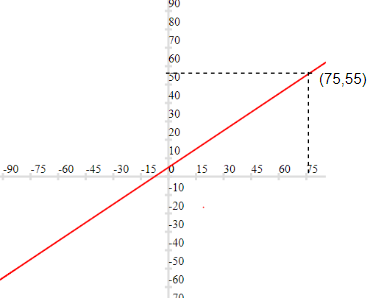Also we can see from the graph, when number of people suffer from other diseases are 75, number of people suffer from asthma are 55
The message is that Smoking in injurious to the health

Question 23
The equation 2x + 5y = 7 has a unique solution, if x, y are
(a) Natural numbers
(b) Positive real numbers
(c) Real numbers
(d) Rational numbers
Solution

Question 24
Find the area of triangle formed between the x axis, y axis and the line(x+y=-7)
(a) 24.5 units
(b) 49 units
(c) 14 units
(d) 21 units
Solution
The graph of the line (x+y=-7) is show below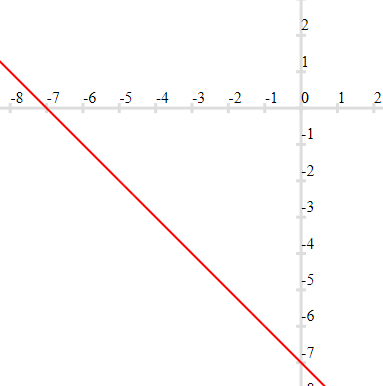We can observe that triangle formed by x axis ,y-axis and this line is a right angle triangle with base 7 units and height 7 units.
So,$Area = \frac {1}{2} 7 \times 7 = 24.5$

Question 25
The graph of y = -1 is a line
(a) parallel to x-axis at a distance 1 units from the origin
(b) parallel to y-axis at a distance 1 units from the origin
(c) making an intercept 1 on the x-axis.
(d) making an intercept 1 on both the axes
Solution
(a)

Question 26
The equation of y-axis is of the form
(a) x = 0
(b) y = 0
(c) x + y = 0
(d) x = y
Solution
(a)

Question 27
Which of the points does not lie on x- axis or y-axis
(a) (10,0)
(b) (10,-10)
(c) (0,1)
(d) (-1,0)
Solution
(b)

## Summary

This Linear equations in two variables class 9 extra questions with solutions is prepared keeping in mind the latest syllabus of CBSE . This has been designed in a way to improve the academic performance of the students. If you find mistakes , please do provide the feedback on the mail. You can download this test as pdf also as belowGo back to Class 10 Main Page using below links

### Practice Question

Question 1 What is $1 - \sqrt {3}$ ?
A) Non terminating repeating
B) Non terminating non repeating
C) Terminating
D) None of the above
Question 2 The volume of the largest right circular cone that can be cut out from a cube of edge 4.2 cm is?
A) 19.4 cm3
B) 12 cm3
C) 78.6 cm3
D) 58.2 cm3
Question 3 The sum of the first three terms of an AP is 33. If the product of the first and the third term exceeds the second term by 29, the AP is ?
A) 2 ,21,11
B) 1,10,19
C) -1 ,8,17
D) 2 ,11,20